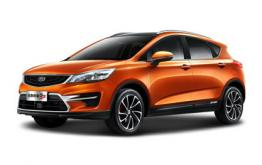吉利汽车 帝豪GS

6.26郑青春 就这Young 帝豪GS深度试驾会等你来嗨

2016年06月21日 11:49 来源：www.chextx.com 超过：8289次关注

你会弹吉他，
你会画手绘，
你会训练自己的狗狗去两条街之外打酱油，
你热爱生活、你满怀期待。
却被古板的上司吆五喝六，
让你觉得世界上难有什么东西可以填补自己内心的万千沟壑。你在办公室里加班加点，
你堵在清晨去公司的路上，
你被别人的无理取闹搞的焦头烂额。
这都是别人嘴里的苟且。
给我们安慰是诗和远方，那里有陶渊明的悠然见南山；
有李白的烟花下扬州；有杜甫的一览众山小。
世界那么美妙，我们何必要画地为牢？束缚你的不是别人制定的条条框框，
不是脚下的横平竖直，
也不是别人对于自己的看法。
而是给自己内心上的一把锁。
倾听灵魂深处发出的余音，
随心所至，
便是最大的自由。6月26日上午10：00-12:00，中州大道河南华驰旗舰店约您来“驾”！
郑青春  就这Young，河南华驰帝豪GS深度试驾会，暨吉利全系120分钟限时抢购会，等你来嗨！吉利汽车厂家独家支持

中州大道河南华驰旗舰店
河南华驰倾力钜献
活动当天：
厂家支持！河南独家！
放肆抄底！限量特价！
多重大礼！幸运大奖！
节目表演！嗨爆到底！
详情咨询：河南华驰销售热线：0371-55655987/60381666 （4000—715--718）

1、 进店礼（活动期间进店即赠价值188元超值大礼包1份）

2、 试驾礼（试驾帝豪GS即赠精美礼品一份）
3、 分期礼（购吉利新帝豪畅享2年0利息，万元钜惠）
4、 置换礼（任意车型置换吉利GX7最高补贴5000元）
5、 订车礼（制定车型购车赠送价值888元行车记录仪）
6、 特价礼（吉利新帝豪限量特价车，最高现金直降12000元！）
吉利GX7 购车首次跌破出厂价，我们只做厂家的搬运工！
………
6月26，中州大道河南华驰店，约您来驾！

省事：一站式搞定您的选车、购车、保险、分期、上牌，提供专业的顾问式购车服务。

省时：紧邻中州大道交通便利不堵车；合格证出证快上牌不担忧；足不出户即可选购你的爱车！
省心：价格一步到位！一降到底！
购车贷款更加“方便、快捷”！
“省心、放心”的一站式保险管家服务
“专业、可靠”的维修保养服务相关文章

0-500 字已有评论 0条 查看评论>>

帝豪GS猜你喜欢

﻿
• 快速找车
• 选择品牌
• 选择品牌
• A  奥迪
• A  阿斯顿·马丁
• A  阿尔法·罗密欧
• B  宝沃
• B  布加迪
• B  巴博斯
• B  保时捷
• B  宾利
• B  奔驰
• B  宝马
• B  本田
• B  别克
• B  标致
• B  比亚迪
• B  宝骏
• B  北汽制造
• B  北汽新能源
• B  北汽幻速
• B  北汽威旺
• B  北京汽车
• B  奔腾
• B  北汽绅宝
• C  长安
• C  长安商用
• C  长城
• C  昌河
• D  大众
• D  道奇
• D  DS
• D  东南
• D  东风风神
• D  东风风行
• D  东风小康
• D  东风风度
• D  东风
• F  福特
• F  丰田
• F  菲亚特
• F  法拉利
• F  福田
• F  福迪
• F  福汽启腾
• G  观致
• G  广汽传祺
• G  广汽吉奥
• G  GMC
• H  红旗
• H  汉腾汽车
• H  哈弗
• H  哈飞
• H  海格
• H  海马
• H  华颂
• H  黄海
• H  华泰
• H  恒天
• J  吉利汽车
• J  捷豹
• J  Jeep
• J  江淮
• J  江铃
• J  金杯
• J  九龙
• J  金旅
• K  凯翼
• K  凯迪拉克
• K  克莱斯勒
• K  科尼塞克
• K  卡威
• K  开瑞
• L  路虎
• L  林肯
• L  劳斯莱斯
• L  兰博基尼
• L  雷克萨斯
• L  铃木
• L  雷诺
• L  理念
• L  力帆
• L  莲花汽车
• L  猎豹
• L  路特斯
• L  陆风
• M  马自达
• M  MG
• M  MINI
• M  玛莎拉蒂
• M  摩根
• M  迈凯轮
• N  纳智捷
• O  欧宝
• O  讴歌
• O  欧朗
• Q  奇瑞
• Q  起亚
• Q  启辰
• R  日产
• R  荣威
• R  瑞麒
• S  三菱
• S  斯威汽车
• S  萨博
• S  smart
• S  斯柯达
• S  斯巴鲁
• S  思铭
• S  双龙
• S  上汽大通
• S  双环
• T  特斯拉
• T  腾势
• W  沃尔沃
• W  五菱汽车
• W  五十铃
• W  威兹曼
• W  威麟
• X  现代
• X  雪佛兰
• X  雪铁龙
• X  西雅特
• Y  一汽
• Y  英菲尼迪
• Y  英致
• Y  依维柯
• Y  野马汽车
• Y  永源
• Z  众泰
• Z  中华
• Z  中兴
• Z  知豆
• 选择车系
• 选择车系
• 车型对比
• 选择品牌
• 选择品牌
• A  奥迪
• A  阿斯顿·马丁
• A  阿尔法·罗密欧
• B  宝沃
• B  布加迪
• B  巴博斯
• B  保时捷
• B  宾利
• B  奔驰
• B  宝马
• B  本田
• B  别克
• B  标致
• B  比亚迪
• B  宝骏
• B  北汽制造
• B  北汽新能源
• B  北汽幻速
• B  北汽威旺
• B  北京汽车
• B  奔腾
• B  北汽绅宝
• C  长安
• C  长安商用
• C  长城
• C  昌河
• D  大众
• D  道奇
• D  DS
• D  东南
• D  东风风神
• D  东风风行
• D  东风小康
• D  东风风度
• D  东风
• F  福特
• F  丰田
• F  菲亚特
• F  法拉利
• F  福田
• F  福迪
• F  福汽启腾
• G  观致
• G  广汽传祺
• G  广汽吉奥
• G  GMC
• H  红旗
• H  汉腾汽车
• H  哈弗
• H  哈飞
• H  海格
• H  海马
• H  华颂
• H  黄海
• H  华泰
• H  恒天
• J  吉利汽车
• J  捷豹
• J  Jeep
• J  江淮
• J  江铃
• J  金杯
• J  九龙
• J  金旅
• K  凯翼
• K  凯迪拉克
• K  克莱斯勒
• K  科尼塞克
• K  卡威
• K  开瑞
• L  路虎
• L  林肯
• L  劳斯莱斯
• L  兰博基尼
• L  雷克萨斯
• L  铃木
• L  雷诺
• L  理念
• L  力帆
• L  莲花汽车
• L  猎豹
• L  路特斯
• L  陆风
• M  马自达
• M  MG
• M  MINI
• M  玛莎拉蒂
• M  摩根
• M  迈凯轮
• N  纳智捷
• O  欧宝
• O  讴歌
• O  欧朗
• Q  奇瑞
• Q  起亚
• Q  启辰
• R  日产
• R  荣威
• R  瑞麒
• S  三菱
• S  斯威汽车
• S  萨博
• S  smart
• S  斯柯达
• S  斯巴鲁
• S  思铭
• S  双龙
• S  上汽大通
• S  双环
• T  特斯拉
• T  腾势
• W  沃尔沃
• W  五菱汽车
• W  五十铃
• W  威兹曼
• W  威麟
• X  现代
• X  雪佛兰
• X  雪铁龙
• X  西雅特
• Y  一汽
• Y  英菲尼迪
• Y  英致
• Y  依维柯
• Y  野马汽车
• Y  永源
• Z  众泰
• Z  中华
• Z  中兴
• Z  知豆
• 选择车系
• 选择车系
• 选择车型
• 选择车型
• 意见反馈